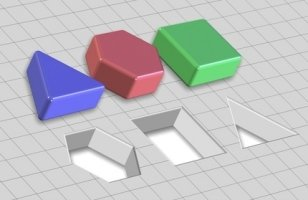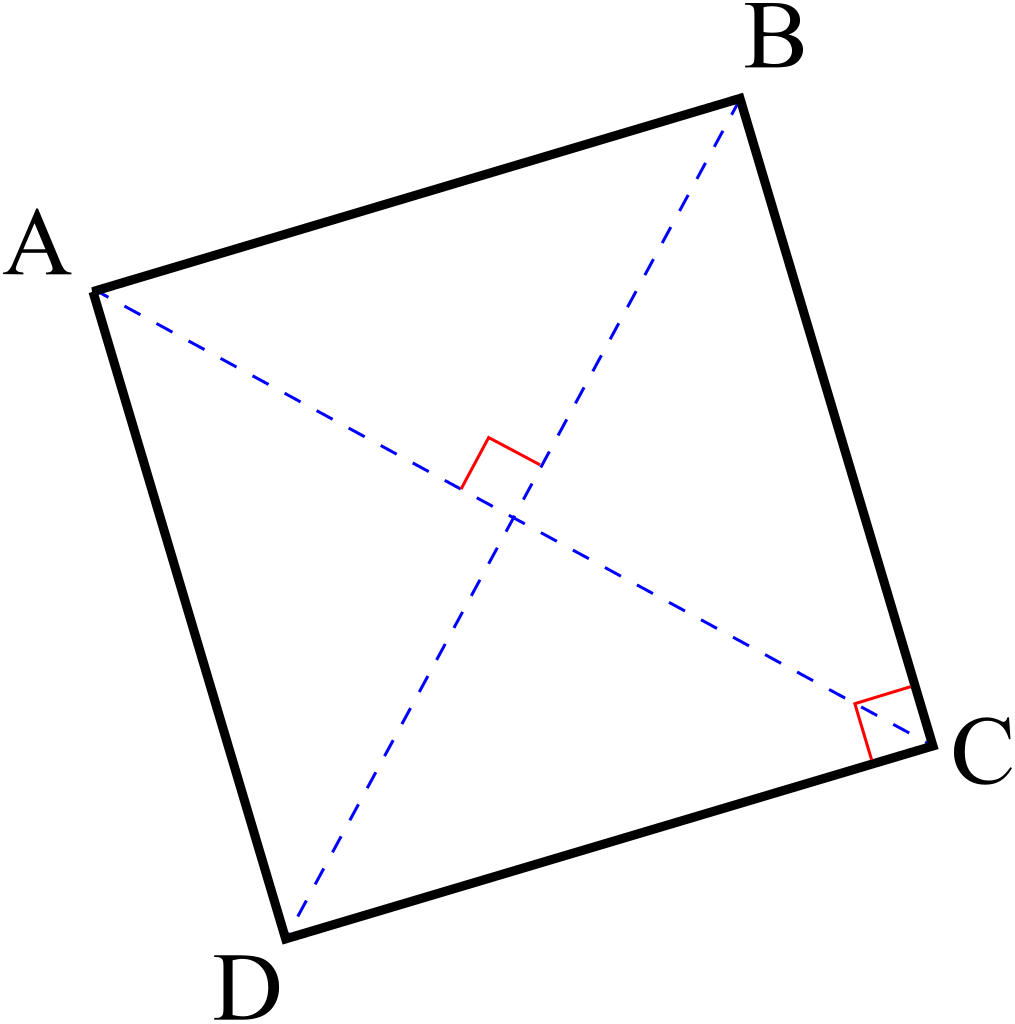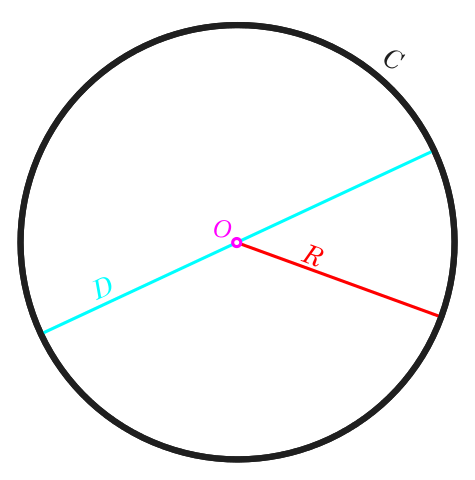## Area converterThis area converter allows you to simultaneously convert an area or a surface to several common units like square metre (base unit of surface in the International System of units - SI), square foot, square kilometre. Enter the area in the second column and the conversion will be performed automatically in all other units.

Common area units :
 square millimetre mm2 square metre m2 hectare ha square kilometre km2 square inch sq in square foot sq ft square yard sq yd square mile sq mi
Less used area units :
 square centimetre cm2 square decimetre dm2 centiare ca square decametre dam2 are a square hectometre hm2 square nautic mile rood ro acre acro lí (China) fen (China) mu (China) shi (China) qing (China) Tsubo (Japan) Cho (Japan) Tann (Japan) Se (Japan) metric Dunam Dunam (Cyprus) Dunam (Iraq) Greek Stemma

## Equivalency between the area and the side of a square

Compute side or diagonal of a square from its surface :area in m2 side AB in metres m diagonal AC in metres m

## Equivalency between the area and the radius of a disk (or wheel)

Compute radius or diameter of a disk (circle) from its area :area in m2 radius in metres m diameter in metres m